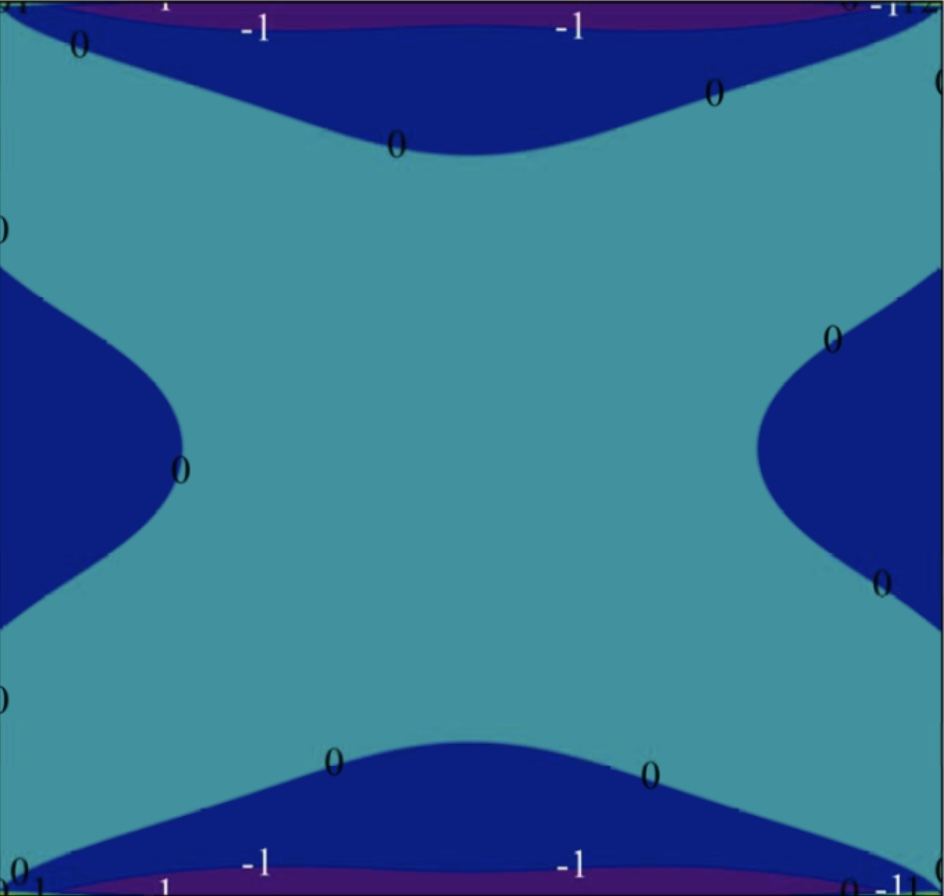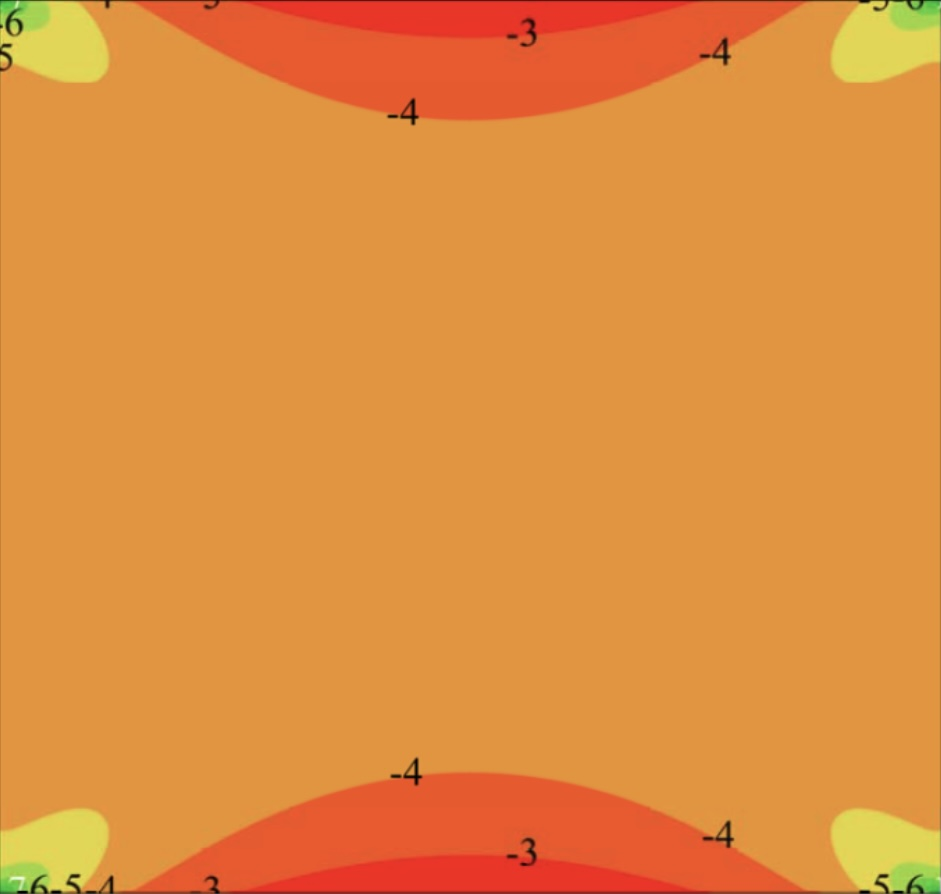# A TEMPERATURE PROBLEM FOR A SQUARE: AN EXACT SOLUTION

Determining the thermal stresses in elastic plates is a necessary component in strength calculations of elements of thin-walled structures, for example, the skin of aircraft and rockets in aerodynamic heating conditions.

Knowledge of the magnitude and nature of the action of thermal stresses is necessary for a comprehensive analysis of the strength of the structure. Thermal stresses in themselves and in combination with mechanical stresses from external forces can cause cracks and destruction of structures made of materials with increased fragility.

In addition, practice shows that thermal stresses can be one of the main causes of rock bursts and, possibly, small-scale earthquakes. This is due to the fact that the heating of the rock and its subsequent cooling lead to the emergence of residual stresses and their subsequent relief in the form of shootings, rock bursts, earthquakes and other catastrophic phenomena.

There are examples of solving the simplest temperature problems in nearly all textbooks on the theory of elasticity. Various methods have been applied for their determination: the superposition method, the method of Fourier integrals, the collocation method, the finite element method, etc. Almost all of them, in one way or another, reduce the boundary value problem to an approximate solution to infinite systems of algebraic equations.

In this work, for the first time, examples of exact solutions to temperature problems for a square with different boundary conditions on its sides are constructed in the following formulations: (1) the temperature problem for a free square; (2) the temperature problem for a clamped square. The solution method is as follows. First, the temperature problem for an infinite plane is solved. Then, exact solutions to homogeneous boundary value problems for a rectangle are added to this solution, with the help of which the boundary conditions on the sides of the square are satisfied. The thermal displacements and stresses are obtained in the form of series in Papkovich–Fadle eigenfunctions. It is shown that in the problem for a free square the tangential stresses have finite spikes at the corner points. There will be no spikes if we assume that the expanded function vanishes in a small neighborhood of the corner points. We can do otherwise by multiplying the expanded function in the neighborhood of the corner points by a smoothing function that vanishes at the corner points together with the given number of its derivatives. It is shown that in the problem for a clamped square the spikes of the normal stresses at the corner points have a logarithmic character. It is impossible to avoid this singularity. This is due to the fact that the Lagrange coefficient for the expanded function has the highest rate of decrease since it is determined directly from the equation for determining biorthogonal functions and, therefore, the expanded function is treated in this case as an entire one, i.e. it possesses the greatest smoothness. Thus, the singularity for the stresses in the temperature problem for a clamped square is its physical characteristic.

To avoid nonprincipal calculations and to make the presentation maximally simple and brief, we consider a square rather than a rectangle whose temperature field is assumed to be even-symmetric relative to the central coordinate axes of the square; the temperature distribution law is chosen to be maximally simple:

T(x,y)=x2 + y2Fig. 1. Contour lines for normal stresses  in a free square.Fig. 2. Contour lines for normal stresses  in a clamped square.

The technique of solving temperature problems can also be applied to solving inhomogeneous problems.

The research was carried out with the support of the Russian Science Foundation (project No. 19-71-00094) and the Russian Foundation for Basic Research in cooperation with the National Natural Science Foundation of China (project No. 20-51-53021).

Source: Kovalenko M.D., Menshova I.V., Kerzhaev A.P., Yu G. A temperature problem for a square: An exact solution // Mathematics and Mechanics of Solids, 2022, vol. 27, no. 2, pp. 250–261. DOI: 10.1177/10812865211020496.